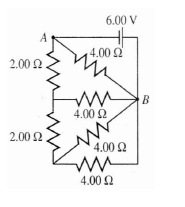# Problem: What is the current through the 4.00-Ω resistor at the bottom given that the equivalent resistance for the entire circuit is 2.00Ω?

###### FREE Expert Solution

Equivalent resistance for resistors in parallel:

$\overline{)\frac{\mathbf{1}}{{\mathbf{R}}_{\mathbf{eq}}}{\mathbf{=}}\frac{\mathbf{1}}{{\mathbf{R}}_{\mathbf{1}}}{\mathbf{+}}\frac{\mathbf{1}}{{\mathbf{R}}_{\mathbf{2}}}{\mathbf{+}}{\mathbf{.}}{\mathbf{.}}{\mathbf{.}}{\mathbf{+}}\frac{\mathbf{1}}{{\mathbf{R}}_{\mathbf{n}}}}$

89% (407 ratings)###### Problem Details

What is the current through the 4.00-Ω resistor at the bottom given that the equivalent resistance for the entire circuit is 2.00Ω?